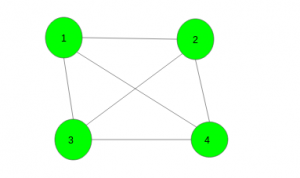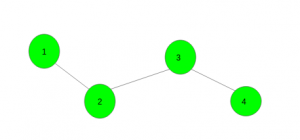# Find K vertices in the graph which are connected to at least one of remaining vertices

Given a connected graph with N vertices. The task is to select k(k must be less than or equals to n/2, not necessarily minimum) vertices from the graph such that all these selected vertices are connected to at least one of the non selected vertex. In case of multiple answers print any one of them.

Examples:

Input :Output : 1
Vertex 1 is connected to all other non selected vertices. Here
{1, 2}, {2, 3}, {3, 4}, {1, 3}, {1, 4}, {2, 4} are also the valid answers

Input :Output : 1 3
Vertex 1, 3 are connected to all other non selected vertices. {2, 4} is also a valid answer.

## Recommended: Please try your approach on {IDE} first, before moving on to the solution.

Efficient Approach: An efficient way is to find vertices which are even level and odd level using simple dfs or bfs function. Then if the verices at odd level are less than the vertices at even level then print odd level vertices. Otherwise, print even level vertices.

Below is the implementation of the above approach:

## C++

 `// C++ program to find K vertices in  ` `// the graph which are connected to at  ` `// least one of remaining vertices ` `#include ` `using` `namespace` `std; ` `#define N 200005 ` ` `  `// To store graph ` `int` `n, m, vis[N]; ` `vector<``int``> gr[N]; ` `vector<``int``> v; ` ` `  `// Function to add edge ` `void` `add_edges(``int` `x, ``int` `y) ` `{ ` `    ``gr[x].push_back(y); ` `    ``gr[y].push_back(x); ` `} ` ` `  `// Function to find level of each node ` `void` `dfs(``int` `x, ``int` `state) ` `{ ` `    ``// Push the vertex in respected level ` `    ``v[state].push_back(x); ` ` `  `    ``// Make vertex visited ` `    ``vis[x] = 1; ` ` `  `    ``// Traverse for all it's child nodes ` `    ``for` `(``auto` `i : gr[x]) ` `        ``if` `(vis[i] == 0) ` `            ``dfs(i, state ^ 1); ` `} ` ` `  `// Function to print vertices ` `void` `Print_vertices() ` `{ ` `    ``// If odd level vertices are less ` `    ``if` `(v.size() < v.size()) { ` `        ``for` `(``auto` `i : v) ` `            ``cout << i << ``" "``; ` `    ``} ` `    ``// If even level vertices are less ` `    ``else` `{ ` `        ``for` `(``auto` `i : v) ` `            ``cout << i << ``" "``; ` `    ``} ` `} ` ` `  `// Driver code ` `int` `main() ` `{ ` `    ``int` `n = 4, m = 3; ` ` `  `    ``// Add edges ` `    ``add_edges(1, 2); ` `    ``add_edges(2, 3); ` `    ``add_edges(3, 4); ` ` `  `    ``// Function call ` `    ``dfs(1, 0); ` ` `  `    ``Print_vertices(); ` ` `  `    ``return` `0; ` `} `

## Java

 `// Java program to find K vertices in  ` `// the graph which are connected to at  ` `// least one of remaining vertices ` `import` `java.util.*; ` ` `  `class` `GFG ` `{ ` ` `  `    ``static` `final` `int` `N = ``200005``; ` ` `  `    ``// To store graph ` `    ``static` `int` `n, m; ` `    ``static` `int``[] vis = ``new` `int``[N]; ` `    ``static` `Vector[] gr = ``new` `Vector[N]; ` `    ``static` `Vector[] v = ``new` `Vector[``2``]; ` ` `  `    ``// Function to add edge ` `    ``static` `void` `add_edges(``int` `x, ``int` `y)  ` `    ``{ ` `        ``gr[x].add(y); ` `        ``gr[y].add(x); ` `    ``} ` ` `  `    ``// Function to find level of each node ` `    ``static` `void` `dfs(``int` `x, ``int` `state)  ` `    ``{ ` `        ``// Push the vertex in respected level ` `        ``v[state].add(x); ` ` `  `        ``// Make vertex visited ` `        ``vis[x] = ``1``; ` ` `  `        ``// Traverse for all it's child nodes ` `        ``for` `(``int` `i : gr[x]) ` `        ``{ ` `            ``if` `(vis[i] == ``0``) ` `            ``{ ` `                ``dfs(i, state ^ ``1``); ` `            ``} ` `        ``} ` `    ``} ` ` `  `    ``// Function to print vertices ` `    ``static` `void` `Print_vertices()  ` `    ``{ ` `        ``// If odd level vertices are less ` `        ``if` `(v[``0``].size() < v[``1``].size())  ` `        ``{ ` `            ``for` `(``int` `i : v[``0``])  ` `            ``{ ` `                ``System.out.print(i + ``" "``); ` `            ``} ` `        ``}  ` `         `  `        ``// If even level vertices are less ` `        ``else`  `        ``{ ` `            ``for` `(``int` `i : v[``1``])  ` `            ``{ ` `                ``System.out.print(i + ``" "``); ` `            ``} ` `        ``} ` `    ``} ` ` `  `    ``// Driver code ` `    ``public` `static` `void` `main(String[] args) ` `    ``{ ` `        ``n = ``4``; ` `        ``m = ``3``; ` `        ``for` `(``int` `i = ``0``; i < N; i++) ` `        ``{ ` `            ``gr[i] = ``new` `Vector(); ` `        ``} ` `        ``for` `(``int` `i = ``0``; i < ``2``; i++)  ` `        ``{ ` `            ``v[i] = ``new` `Vector(); ` `        ``} ` `         `  `        ``// Add edges ` `        ``add_edges(``1``, ``2``); ` `        ``add_edges(``2``, ``3``); ` `        ``add_edges(``3``, ``4``); ` ` `  `        ``// Function call ` `        ``dfs(``1``, ``0``); ` ` `  `        ``Print_vertices(); ` `    ``} ` `} ` ` `  `// This code is contributed by 29AjayKumar `

## Python3

 `# Python3 program to find K vertices in ` `# the graph which are connected to at ` `# least one of remaining vertices ` ` `  `N ``=` `200005` ` `  `# To store graph ` `n, m, ``=``0``,``0` `vis``=``[``0` `for` `i ``in` `range``(N)] ` `gr``=``[[] ``for` `i ``in` `range``(N)] ` `v``=``[[] ``for` `i ``in` `range``(``2``)] ` ` `  `# Function to add edge ` `def` `add_edges(x, y): ` `    ``gr[x].append(y) ` `    ``gr[y].append(x) ` ` `  `# Function to find level of each node ` `def` `dfs(x, state): ` ` `  `    ``# Push the vertex in respected level ` `    ``v[state].append(x) ` ` `  `    ``# Make vertex visited ` `    ``vis[x] ``=` `1` ` `  `    ``# Traverse for all it's child nodes ` `    ``for` `i ``in` `gr[x]: ` `        ``if` `(vis[i] ``=``=` `0``): ` `            ``dfs(i, state ^ ``1``) ` ` `  ` `  `# Function to prvertices ` `def` `Print_vertices(): ` ` `  `    ``# If odd level vertices are less ` `    ``if` `(``len``(v[``0``]) < ``len``(v[``1``])): ` `        ``for` `i ``in` `v[``0``]: ` `            ``print``(i,end``=``" "``) ` `    ``# If even level vertices are less ` `    ``else``: ` `        ``for` `i ``in` `v[``1``]: ` `            ``print``(i,end``=``" "``) ` ` `  `# Driver code ` ` `  `n ``=` `4` `m ``=` `3` ` `  `# Add edges ` `add_edges(``1``, ``2``) ` `add_edges(``2``, ``3``) ` `add_edges(``3``, ``4``) ` ` `  `# Function call ` `dfs(``1``, ``0``) ` ` `  `Print_vertices() ` ` `  `# This code is contributed by mohit kumar 29 `

Output:

```2 4
```

Time Complexity : O(V+E)
Where V is the number of vertices and E is the number of edges in the graph.

My Personal Notes arrow_drop_uppawanasipugmailcom

If you like GeeksforGeeks and would like to contribute, you can also write an article using contribute.geeksforgeeks.org or mail your article to contribute@geeksforgeeks.org. See your article appearing on the GeeksforGeeks main page and help other Geeks.

Please Improve this article if you find anything incorrect by clicking on the "Improve Article" button below.

Article Tags :
Practice Tags :

Be the First to upvote.

Please write to us at contribute@geeksforgeeks.org to report any issue with the above content.